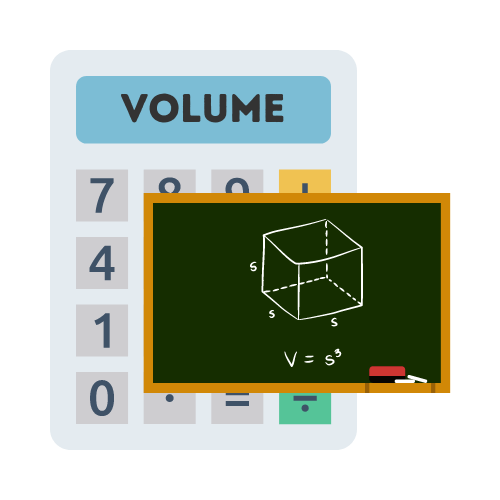# Volume Calculator

## Calculate the volume of a cube, cylinder or sphere

• Created by John Harris
• Reviewed by Ramesh Agarwal

The volume is:

## What is the volume in geometry?

Volume refers to the amount of space which is occupied by an object. It is the three dimensional counterpart of the two dimensional concept of area. Volume is usually quantified in three dimensional space, and is represented by the symbol V. The cube has the largest volume of any other cuboid.

## How to calculate the volume of a cube?

We can calculate the volume of a cube with the formula `V = L x W x H`. We simply need to measure each side of the cube and then multiply all the values together to get the total cube volume. The cube is one of the easiest formulas to remember when calculating the volume of geometric shapes.

## How to calculate the volume of a cylinder?

The term cylinder refers to the shape of the object. It has a circular base and a cylinder that extends from the base. The base and elongated body of the object can be of the same length and height. Sometimes the cylinder shape is known as a solid cylinder. However, it is also used to describe a hollow object (tube).

The volume of a cylinder is equal to the area of the base times the height. The formula for the volume of a cylinder is `V = πr2h`. We need to multiply Pi by the radius squared, then multiply the result by the height of the cylinder. An easier way is to use a volume of a cylinder calculator like the one on this page. Simply enter the measurements and let the calculator do the rest.

## How to calculate the volume of a sphere?

We can calculate the volume of a sphere by using the formula `V = 4/3 πr3`. We can break this down further by dividing 4 by 3, then multiplying by Pi, then multiplying the result by the sphere's radius cubed. An easier way to get the sphere's volume is to use a volume of a sphere calculator.

## Why do we calculate the volume in geometry?

A geometric shape is a three-dimensional object with length, width and height. The volume of a shape is the amount of space inside a given shape. The main reason we calculate the volume of a shape, is to find out how much space it occupies.

Volume is the amount of space contained within an object. To calculate the volume of a shape, we first look at the shape's dimensions in order to determine the correct formula. The cylinder volume formula is different from the sphere volume formula or the formula for cube volume. The first step is to determine what shape we are working with.

## What is a real world use for calculating volume?

The volume of objects is important in physics for a number of reasons. One example is the affects of gravity. If we know the volume of an object, then we can calculate the gravitation pull on the object, or to put it more simply: how fast it will fall to the ground if dropped.

Calculating volume becomes important in freight shipping. When transporting goods via sea or air, there is limited space and therefore the larger volume that your goods take up the more the cost of transport will be.

Contrary to popular belief, the volume is more important than the weight of the goods, due to limited space (volume) of the vessel.

## FAQS

To find the volume of a cube, you raise the length of one side (s) to the power of 3. The formula is V = s³, where V is the volume of the cube and s is the length of one side.

The formula for finding the volume of a cylinder is V = πr²h, where V is the volume, r is the radius of the base, and h is the height of the cylinder.

The formula for finding the volume of a sphere is V = (4/3)πr³, where V is the volume and r is the radius of the sphere.

Yes, you can use the diameter (d) to find the volume of a sphere. First, divide the diameter by 2 to get the radius, and then use the formula V = (4/3)πr³ to find the volume.

The value of π (pi) is approximately 3.14159, but it is an irrational number with infinite decimal places.

If you know the length of one side (s) of a cube, you can use the formula V = s³ to find its volume.

No, the circumference is not used to find the volume of a cylinder. You need to know the radius (or diameter) and height to calculate the volume.

The units for volume depend on the units used for the measurements. For example, if the length is in meters, the volume will be in cubic meters (m³).